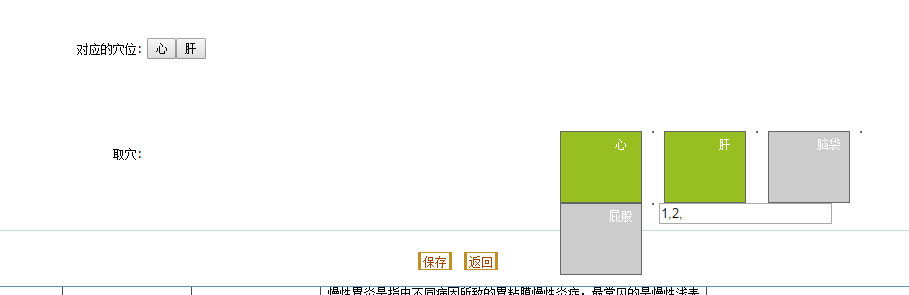**

``````
var arr1 = [ { "name" : "心", "acupoint_num" : "1" }, { "name" : "肝",
"acupoint_num" : "2" }, { "name" : "脑袋", "acupoint_num" : "3" }, { "name" : "屁股",
"acupoint_num" : "4" } ];

var retStr = ",";
\$('#xueweiList').append(
\$(arr1).map(
function() {
return '<li id=' + this.acupoint_num
+ '><a href="#" >' + this.name
+ '</a></li><li>'
}).get().join(''));
\$("#nav>ul>li").click(
function() {
var focus = \$(this).toggleClass('h_nav_over')
.hasClass('h_nav_over');
if (focus)
retStr += this.id + ',';
else
retStr = retStr.replace(',' + this.id + ',',
',');

\$("#xueweis").val(retStr.replace(/^,|,\$/g, ''));
});

/* 2-获取疾病对应穴位 */
var arr2 = [ { "earName" : "心", "acupoint_num" : "1" }, { "earName" : "肝",
"acupoint_num" : "2" } ];
var arrStr2 = '';
var xueweisStr = "";
\$.each(arr2, function(index2, item) { // 获取后台传来的json是数组
arrStr2 += "<input type='button' id='"
+ arr2[index2].acupoint_num + "' value='"
+ arr2[index2].earName + "' name='earName'/>";
\$.each(arr1, function(index, item) {
if(arr2[index2].acupoint_num==arr1[index].acupoint_num){
\$("#"+arr1[index].acupoint_num).css({"background-color":"#98bf21"});
\$("#xueweis").val(xueweisStr);//直接用js返回对应的穴位值给inupt赋值

}
});

xueweisStr += arr2[index2].acupoint_num + ",";

});

\$("#xueweis").val(xueweisStr);//直接用js返回对应的穴位值给inupt赋值
var td2 = document.getElementById("xueweiTd2");
var div2 = document.getElementById("xuewei2");
div2.innerHTML = arrStr2;
td2.appendChild(div2);

});
``````2个回答

retStr你不是没有赋值，要依据arr2的值给retStr赋值

``````  \$.each(arr2, function (index2, item) { // 获取后台传来的json是数组
retStr +=item.acupoint_num+ ",";///////////////
//////........
``````bulidfer 还是没弄出来 就差最后整合这一步了 后台传的值 和前端修改的值bulidfer 你当时赋值是一个逗号，bulidfer 大侠 我都晕了 这个问题已经弄了快两周了 我试试bulidfer 点击以后对arr1 返回的值 没有改变bulidfer 改成retStr后 arr1返回的心 和肝 成死的了 怎么点都不会消除bulidfer 改成retStr了 还是不行